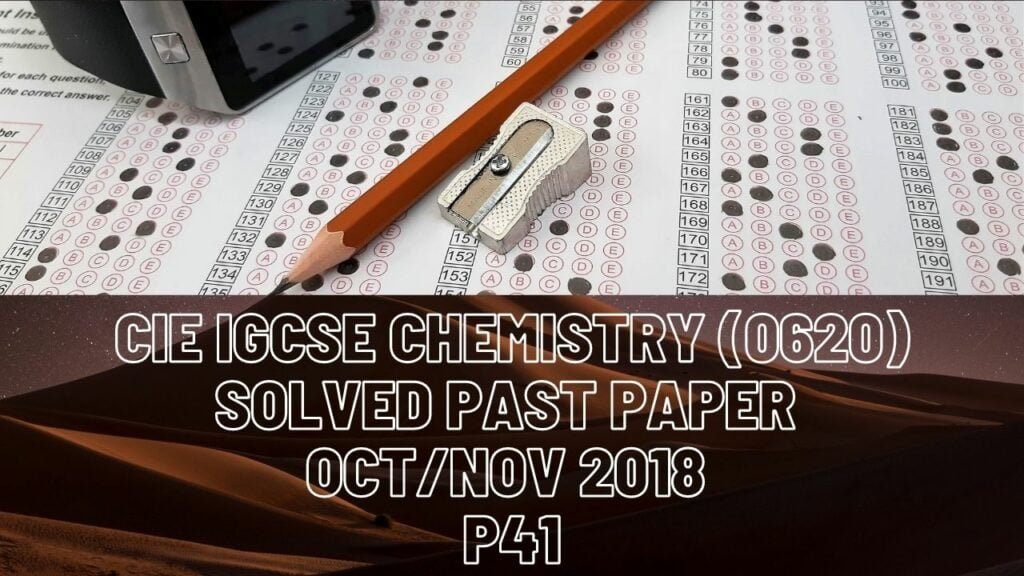IGCSE Chemistry### CIE IGCSE Chemistry Solved Past Paper Oct/Nov 2018 P41

1a) Aluminium

b) Calcium carbonate

c) Methane

d) Sulfur dioxide

e) Chlorine

f) Methane

2a) B-number of electrons is 11

C-number of protons is 18

D-electronic structure-2,8,8 charge is -1

b) A and B are isotopes

c) An element that is above A (A is sodium, protons number-11.Reactivity

of elements in group 1 increases going down the group)

d) It has a complete outer shell of 8 electrons, and so it does not need to react

3a(i) 2Cu(NO)3 —–> O2+4NO2+2CuO

b) At very high temperatures, nitrogen and oxygen react together

c(i) Moles=mass/molar mass

molar mass of copper(ll)nitrate = 64+(2*14)+(6*16) = 188

moles=18.8/188 = 0.1 moles

(ii) From the equation: 2 mole of Cu(NO3)2 give 1 mole of oxygen

so oxygen mole = 0.1/2 = 0.05 mol

(iii) Volume = moles * molar volume

= 0.05*2400 = 1200cm3

d(i) Cu(OH)2

(ii) Zinc is more reactive than copper, so it displaces copper to form zinc nitrate. Zinc nitrate is colourless, and the brown solid is copper metal

(ii) 1-sodium hydroxide 2-aluminium (reagents for test of nitrate ion)

e(i) CuCO3+2HNO3 ——> Cu(NO3)2+H2O+CO2

(II) Respiration

(III) Photosynthesis

4a(i) Fossil fuel

(ii) Contact process

temperature of 450 degrees Celcius

pressure of 1 atmospheric pressure

b(i) Water

(ii) Carbon

c(i) Loss of electrons

(ii) Sulfur single bonded to each of the two hydrogen atoms

(iii) To boil hydrogen sulfide, its intermolecular forces or the forces between its molecules have to be broken. As these forces are weak for hydrogen sulfide, its boiling point is low

d(i) Moles= concentration*volume

0.15*(20/1000)=3*10^-3

(ii) Use ratio set in equation to derive molar value

moles=(3*10^-3)*2

(iii) Volume=moles/concentration

= (6*10^-3)/0.2

= 0.03dm3*1000 =30cm3

5a) Rate of forward reaction is equal to rate of backward reaction,

concentration of reactants and products remains the same

b(i) Equal number of molecules on both side means change in pressure doesn’t change position of equilibrium

(ii) Forward reaction is exothermic, as low temperature gives higher yield

c(i) At the beginning(with steepest part of curve)

d(i) Rate of collisions increases as more particles are available per unit volume so rate of reaction also increases

(ii) Rate of reaction increases, as molecules gain kinetic energy and move faster. Greater proportion of molecules has energy>activation energy, and rate of collisions also increases

6a(i) A compound that consists of carbon and hydrogen atoms only

(ii) CnH2n+2  CnH(2n+1)OH

(ii) They have the same functional group and trends in their physical properties

b(i) Ultraviolet light

(iii) Hydrogen chloride

c) Propyle ethanoate

d(i) C5H10O2

(ii) Methanoic acid, and butan-2-ol, with OH group on second carbon in the chain.